International Journal of Science, Technology and Society
Volume 3, Issue 4, July 2015, Pages: 183-190

Experimental and Numerical Study of Free Convective Heat Transfer on the Triangular Fin for the Optimum Condition of Gap's Configuration

Goshayeshi Hamid Reza, Hashemi Bahman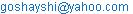(G. H. Reza)

Goshayeshi Hamid Reza, Hashemi Bahman. Experimental and Numerical Study of Free Convective Heat Transfer on the Triangular Fin for the Optimum Condition of Gap's Configuration. International Journal of Science, Technology and Society. Vol. 3, No. 4, 2015, pp. 183-190. doi: 10.11648/j.ijsts.20150304.22

Abstract: In this study, the effect of different conditions for making gap in the triangular fin has been investigated on the free convective heat transfer coefficient. Making gap in suitable places on the monolith triangular fins can increase the free convective heat transfer coefficient, so the purpose of this study is to reach the optimum condition of configuration for gaps by which the free convective heat transfer coefficient is increased. For this purpose, eight different conditions of vertical triangular fins located on a vertical plate were made and investigated. One of these conditions doesn't have gap and the other seven conditions had different kinds of gaps configuration. The obtained results showed that the optimum condition is a condition with the configuration of three gaps on the length of the triangular fin. Also for validation of experimental results, the numerical modeling of all the above mentioned conditions was done by using the fluent software.

Keywords: Triangular Fin, Optimum Configuration of Gaps, Monolith Triangular Fin

Contents

1. Introduction

Heat transfer is utilized in various industries, cooling and heating systems and controlling desirable condition in various machines such as electronic devices. Hence, studying heat transfer, especially free convective heat transfer in various industrial fields and natural processes are of significance. In fact, the need for decreasing function temperature without using forced convection has resulted in carrying out studies to find free convection improving methods and free convective heat transfer in developed surfaces has been a topic to many laboratory and theoretical studies. In 1963, Starner and McManus  studied the free convective heat transfer for four various heat sink models in various dimensions and distances between fins on vertical base surface. In 1965, Welling and Wooldridge  carried out laboratory experiences on angled rectangular heat sinks with constant length which were on vertical surface and calculated the fins optimal height for making the highest heat transfer ratio. In 1985, Aziz  studied the heat optimization and heat transfer ratio in triangular and rectangular fins under convective boundary conditions.

In 1986, Jofre and Baron  studied the free convective heat transfer by air on vertical surface with triangular groove nesses. In 1988, Kondepudi and O’Neal  studied the triangular acicular fins performance under dew layer condition. In 1991, Leung  studied the optimal distance for conical fins arrangement and indicated that this distance is relatively independent of the fins arrangement. In 1995, Abratet and Newnham  analyzed the triangular fins attached to a thick wall by finite element method. In 1998, Kordyban  compared the performance of heat sinks with direct fins and acicular fins. Results to his research suggested that the performance of the heat sink with acicular fins was significantly better than heat sink with direct fins. In 2009, Kobus and Oshio  came to this conclusion that the heat performance of an acicular fin heat sink is a weak function of fin diameter and it increases by the increase in fin length. Also, acicular fins have the best heat performance when their diameter and height are not equal. In 2007, Nada  carried out a laboratory experiment on horizontal and vertical rectangular finned chambers with fins perpendicular to the chamber surface. He came to this conclusion that the optimal fins distance could be reached for the case that the heat transfer ratio and its performance are maximum. Using numerical modeling in 2008, Edlabadkar et al.  studied the effect of angle change in V-shaped fin rays on free convective heat transfer coefficient. They came to this conclusion that, among all studied angles, 90 degree angle had the highest free convective heat transfer coefficient. In the same year, to predict the triangular fins mass and heat transfer, Barman and Debnath , Rao  and Ji proposed an analytical method. In 2012, Naseriyan and Fahiminiya  studied the free convective heat transfer on V-shaped fins and optimal fins arrangement. Research results suggested that, initially, by the increase in fins distance, the heat resistance decreases until it reaches the optimal distance, which has the lowest heat resistance. By the further increase in distance, heat resistance increases. Also, with equal fin height length and constant temperature difference, free convective heat transfer coefficient increases by the increase in fin distance until it reaches the maximum point. Subsequently, by the further increase in distance, heat transfer coefficient decreases.

2. Free Convective Heat Transfer General Equations

Continuity equation and momentum equation in x direction are as following: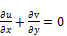(1)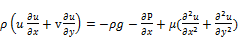(2)

By substituting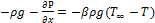in equation (2) and simplification, momentum equation in x direction turns to: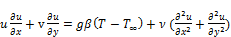(3)

In which,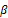is the fluid volumetric thermal expansion coefficient.

In equation (3), it is clear how the buoyancy force, which forms the flow, is related to the temperature difference. Also, momentum equation in y direction is as following: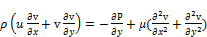(4)

To simultaneous solution of equations (1) and (3) for determination of (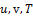), energy equation as the following, is needed: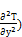(5)

3. Discussion and Results Review

Manufactured triangular fin heat sinks include 8 positions and each heat sink has two fin rows. One of the 8 positions is the position without gaps and with monolith vertical fin, which is considered as the base position (Position 0) and the other 7 positions include various gaps arrangement. The data on fins and base wall dimensions for all manufactured heat sinks is presented in Table (1). Moreover, the distance between the fin rows in each heat sink is 7.9 mm and the angel between fin and base wall in each row is 90 degrees. Figure (1) presents all manufacture triangular fin positions.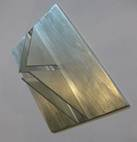Position (3)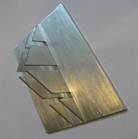Position (7)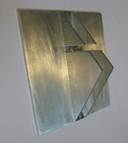Position (2)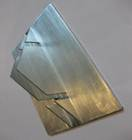Position (6)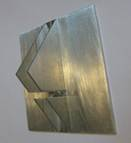Position (1)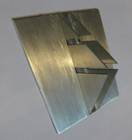Position (5)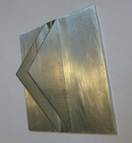Position (0)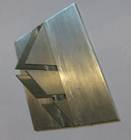Position (4)

Figure 1. All manufacture triangular fin positions.

Table 1. Fins and Base Wall dimensions.

 Base Wall dimensions (mm) Fins dimensions (mm) Length (L) Width (W) Thickness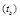Length (L) Height (H) Thickness (t) Gap's Length (S) 80 59.8 1.4 80 20 1 4

The experiments and numerical modeling for all 8 positions were carried out at two temperature differences of 70 to 100 Kelvin, and the results are presented in Diagram (1) and (2). Diagram (1) is related to experimental results and Diagram (2) is related to fluent results in the aforementioned temperature differences.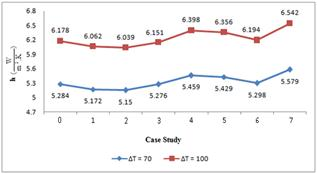Diagram 1. Experimental results at two temperature differences of 70 to 100 Kelvin.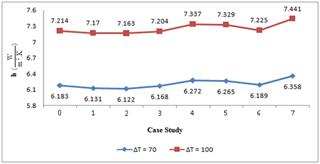Diagram 2. Fluent results at two temperature differences of 70 to 100 Kelvin.

As it could be observed in diagram (1), it is obvious that the experimental results in the two temperature differences are quite similar. This similarity could also be observed in Diagram (2), for results from Fluent software in two temperature differences. Hence, to prevent the confusion, the results from the temperature difference of 70 Kelvin is going be studied. Also, on diagram (1) and (2), it could be observed that by the increase in temperature difference, free convective heat transfer coefficient increases, for by the increase in temperature difference, Grashof number increases; that is, the buoyancy force which creates free convective flow increases and this leads to increase in flow rate and as a result free convective heat transfer coefficient increases. To verify the experimental results from the 8 positions, the experimental results were compared to the results from Fluent software in diagram (3). As it could be observed from diagram (3), the experimental results comply with the results from Fluent software. Diagram (4), present ratio of the experimental amounts of free convective heat transfer coefficient for the 7 gapped positions to the experimental amount of free convective heat transfer coefficient for the position without gap (monolith fin), which is considered as the base position (Position 0). Diagram (5), present ratio of the Fluent amounts of free convective heat transfer coefficient for the 7 gapped positions to the Fluent amount of free convective heat transfer coefficient for the position without gap (monolith fin), which is considered as the base position (Position 0).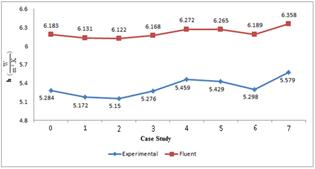Diagram 3. Comparison of experimental results with results from Fluent at temperature difference of 70 Kelvin .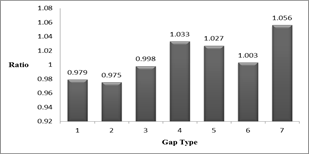Diagram 4. Ratio of the experimental amounts of free convective heat transfer coefficient for the 7 gapped positions to the experimental amount of free convective heat transfer coefficient for the position without gap at temperature difference of 70 Kelvin.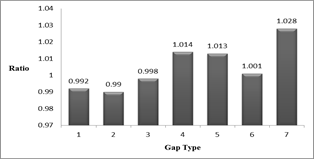Diagram 5. Ratio of the Fluent amounts of free convective heat transfer coefficient for the 7 gapped positions to the Fluent amount of free convective heat transfer coefficient for the position without gap at temperature difference of 70 Kelvin.

In all studied heat sinks, by the increase in distance from the base surface initial edge, the convective heat penetration in free flow increases and the thermal boundary layers grow in two transverse and longitudinal directions of the base surface, which leads to a decrease in free convective heat transfer coefficient. Also, the thermal boundary layers which are not able to grow unlimitedly in the space between the two fins, crash into each other and develop. Thermal boundary layers development also has a remarkable effect on decreasing the free convective heat transfer coefficient, for this prevents establishing a proper free air flow in the space between the fins and as a result, the temperature steep decreases and by the decrease in the temperature steep, free convective heat transfer coefficient and also convective heat transfer ratio decrease. Forming gaps in proper locations on the fins could lead the air to properly flow between the fins and by merging the air between the fins in gaps places with free air flow, the thermal boundary layer thickness decreases and as a result the boundary layer development weakens or even in some cases it is lost, which in turn, it increases the free convective heat transfer coefficient.

Considering the diagram (4) and (5), it is obvious that positions (1) , (2) , and (3) have a relatively lower free convective heat transfer coefficient comparing to monolith fins position. Hence, in studied heat sinks, forming only one gap on the fins is not helpful, for it does not have a huge impact on decreasing thermal boundary layers thickness in two fins outer space and also, lack of ability in preventing thermal boundary layers development or lack of ability in decreasing the development effects formed in two fins outer space. Hence, forming only one gap on the fins not only does not impact the increase in free convective heat transfer coefficient comparing to the monolith fins position, but also it decreases the free convective heat transfer coefficient due to the omission of the useful fins parts which could lay in the thermal boundary layer outer space and exchange heat with the environment air. Also, experimental and Fluent results for position (6) suggest that the increase in free convective heat transfer coefficient for this position is not much sensible and forming gap on the monolith fins under this position arrangement does not provide a huge difference on free convective heat transfer coefficient, comparing to the monolith fins position. That is due to the fact that in position (6), despite the presence of two gaps on fins length, since the distance between these two gaps is huge, thermal boundary layers have the opportunity to remarkably grow after the first gap and before reaching the second gap. Hence, in addition to the fact that the thermal boundary layersin the two fins outer space increases in thickness, the thermal boundary layers in the two fins outer space become completely developed, so that the formation of the second gap is hugely ineffective and cannot decrease the thermal boundary layers effectively and also, leads to a decrease in development effects in the space between the two fins. Accordingly, forming a gap on the monolith fins under this position arrangement is not helpful.

From diagram (4) and (5), it is obvious that positions (4), (5), and (7) have a higher free convective heat transfer coefficient comparing to monolith fins position. Hence, forming gaps on monolith fins under aforementioned positions could lead to an increase in free convective heat transfer coefficient. Due to the continues and close effects which have on the thermal boundary layers, presence of two gaps on the fins length (Positions 4 and 5) could have a great impact on the decrease in boundary layers thickness, delaying their development, or decreasing their development effects and as a result the increase in free convective heat transfer coefficient. The impact could be highly decreased, especially if one of the gaps is formed before reaching the fins half-length (Position 4), for this prevents the excessive growth of thermal boundary layers thickness in fins initial length, where the thermal boundary layer thickness is low, and thermal boundary layers do not have a high thickness while reaching the second gap. As a result, the development of thermal boundary layers happen in higher longitudinal distance from the base surface initial edge. Ultimately, by forming three gaps in fins length, air properly flows between the fins and as a result by merging the air between the fins with free air flow in gaps places, the thermal boundary layers thickness decreases remarkably. This fact leads to a delay in thermal boundary layers development and also effective decrease of its impacts and as a result free convective heat transfer coefficient increases to the maximum. Hence, the best arrangement for forming gaps in a heat sink with triangular fins is the arrangement with three gaps in fins length (Position 7). Figure (2) , (3) and (4) , present the free convective heat transfer coefficient contours for monolith fins (Position 0), the worst gaps arrangement (Position 2) and position (6) are respectively. Also, Figure (5) , (6) , and (7) present the free convective heat transfer coefficient contours for position (4) , (5) and position (7) which has the best gap arrangement, respectively.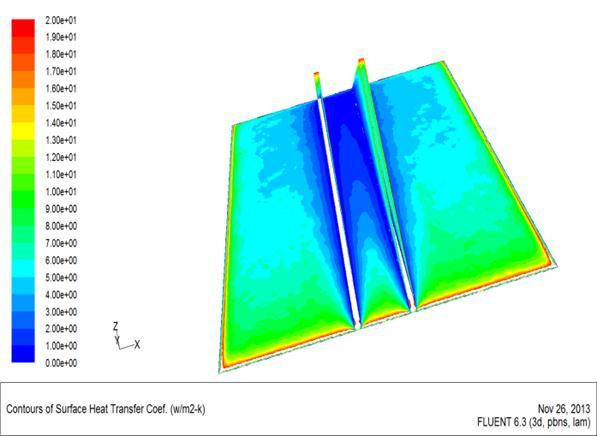Figure 2. Contour of free convective heat transfer coefficient for monolith fin.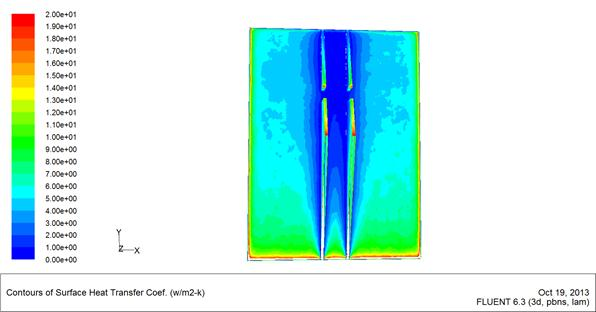Figure 3. Contour of free convective heat transfer coefficient for position 2.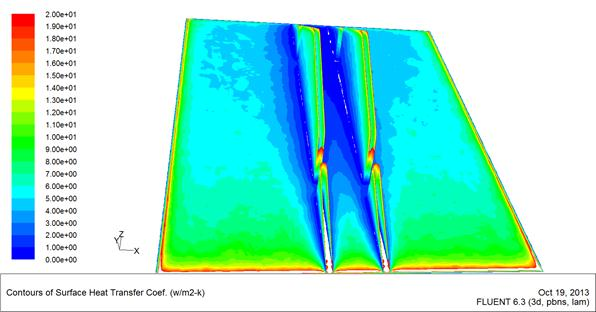Figure 4. Contour of free convective heat transfer coefficient for position 6.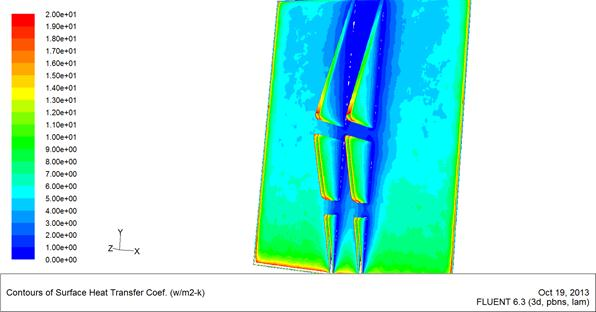Figure 5. Contour of free convective heat transfer coefficient for position 4.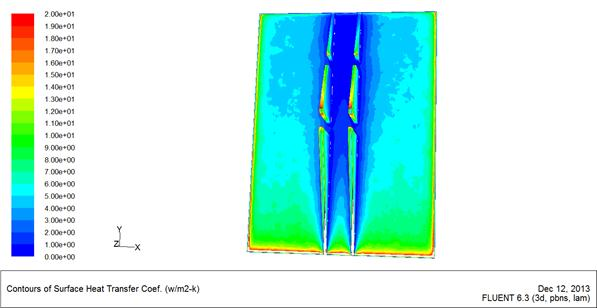Figure 6. Contour of free convective heat transfer coefficient for position 5.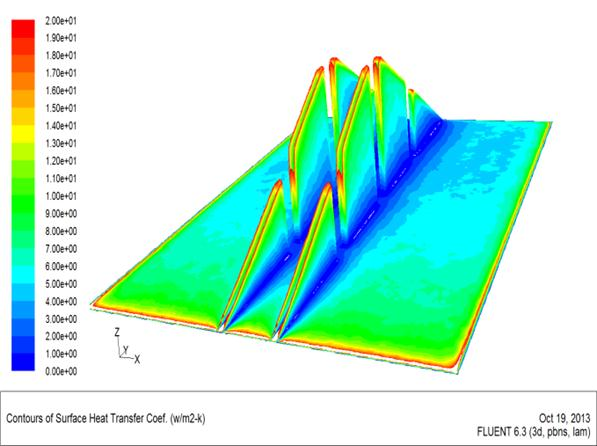Figure 7. Contour of free convective heat transfer coefficient for position 7.

4. Conclusion

By the increase in the distance from the base surface initial edge inheat sinks with vertical fins, the convective heat penetration in free flow increases and the thermal boundary layers grow. By the increase in thermal layer boundary layers thickness and development formation, the temperature steep decreases and by the decrease in the temperature steep, free convective heat transfer coefficient and also convective heat transfer ratio decrease. By forming proper gaps in heat sink fins, the thermal boundary layer development decreases. Forming gaps in proper locations on the fins could lead the air to properly flow between the fins and by merging the air between the fins in gaps places with free air flow, the thermal boundary layer thickness decreases and as a result the boundary layer development weakens or even in some cases it is lost, which in turn, it increases the free convective heat transfer coefficient. The best arrangement for forming gaps in a heat sink with triangular fins is the arrangement with three gaps in fins length (Position 7).

References

1. Starner, K.E., McManus, H.N. (1963) , "An experimental investigation of free convectionheat transfer from rectangular fin arrays", J., Heat Transfer 85(2), pp. 273–278.
2. Welling, J.R., Wooldridge, C.B. (1965) , "Free convection heat transfer coefficientsfrom rectangular vertical fins",Trans. ASME J. Heat Transfer 87 (3), pp.439–444.
3. Aziz, A. (1985) ," OPTIMIZATION OF RECTANGULAR AND TRIANGULAR FINS WITH CONVECTIVE BOUNDARY CONDITION," INT. COMM. HEAT MASS TRANSFER, Vol. 12, pp. 479-482.
4. Jofre, R.J., and Baron, R.F. (1986) , "Free convection heat transfer to a rough plate," ASME Paper No. 67.
5. Kondepudi, S. N., O'Neal, D. L. (1988) , "PERFORMANCE OF TRIANGULAR SPINE FINS UNDER FROSTING CONDITIONS," Heat Recovery Systems & CHP. Vol. 8, No.1, pp. 1-7.
6. Leung, S. E., Ho, C. W., and Probert, S. D. (1991) , "Performances of heat exchangers with tapered fins".
7. Abratet, S., Newnham, P. (1995) , "FINITE ELEMENT ANALYSIS OF TRIANGULAR FINS ATTACHED TO A THICK WALL," Computers & Structures, Vol. 51, No.6, pp. 945-957.
8. Kordyban, T. (1998) , "Hot air rises and heat sinkeverything you know about cooling electronice is wrong," ASME Press.
9. Kobus, C.J., and Oshio, T. (2004) , "Development of a theoretical model for predicting the thermal performance characteristics of a vertical pin-fin array heat sink under combined forced and natural convection with impinging flow," International Journal of Heat Mass Transfer 48, 1053-1063.
10. Nada, S.A. (2007) ," Natural Convection Heat Transfer in Horizontal and Vertical Closed Narrow Enclosures with Heated Rectangular Finned Base Plate", Int. J. Heat Mass Transfer, Vol. 50, pp. 667-679.
11. Edlabadkar, R.L., Sane, N.K., Parishwad, G.V. (2008) ,"Computational Analysis of Natural Convection With Single V-Type Partition Plate", 5th European Thermal-Sciences Conference, Netherlands.
12. Kundua, B., Barman, D., Debnath, S. (2008) , " An analytical approach for predicting fin performance of triangular fins subject to simultaneous heat and mass transfer," International journal of refrigeration 31, pp. 1113–1120.
13. Rao, V. D., Naidu, S.V., Rao, B.G., Sharma, K.V.,(2006) "Heat Transfer from a Horizontal Fin Array by Natural Convection and Radiation a Conjugate Analysis", Int. J. Heat and Mass Transfer. Vol. 49, pp. 3379–3391.
14. Ji, S.Y. Kim,S, Y, (2007) "Pressure drop and heat transfer correlations for triangular folded fin heat sinks", IEEE Trans. Compon. Packaging Technol. 30 (1).(2007) 3–8.
15. Naserian, M.M., Fahiminia, M., Goshayeshi, H.R. (2013) , "Experimental and numerical analysis of natural convection heat transfer coefficient of V-type fin configurations " Journal of Mechanical Science and Technology.

 Contents 1. 2. 3. 4.
Article ToolsAbstractPDF(828K)